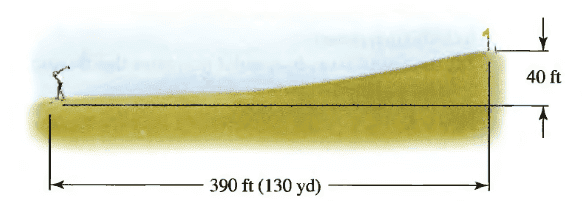# Vector Projectile Problem (Calculus III)

FallingMan

## Homework Statement

A golfer stands 390 ft (130 yards) horizontally from the hole and 40 feet below the hole. Assuming the ball is hit with an initial speed of 150 ft/s, at what angle should it be hit to land in the hole? Assume the path of the ball lies in a plane## Homework Equations

Force of gravity:
a(t) = <0, -32>
32 feet/sec^2 will be the force of gravity)

Integrating a(t) gives us v(t), the velocity function.

Velocity function:
V(t) = <0, -32t> + <Initial Velocity in X Direction, Initial Velocity in Y Direction>

## The Attempt at a Solution

1. I obtain the vector valued function for the velocity of the ball. Let's call that v(t). We know that after the ball is launched, there are no forces acting on the ball in the x-direction, but there is gravity acting on the ball in the y-direction. Thus, the y-component of the vector will be -32t, and the x-component will be 0. The initial velocity vector will be the x and y-components of the initial speed of 150 ft/sec, which will be 150cos(θ), and 150sin(θ), respectively.

v(t) = <0, -32t> + <150cos(θ), 150sin(θ)>
v(t) = <150cos(θ), 150sin(θ)-32t>

2. I obtain the vector valued function for the position of the ball by integrating the velocity function. Let's call the position function p(t):

p(t) = <150sin(θ), -150cos(θ)-16t^2> + <Initial Position on X-Coord, Initial position on Y-Coord>

Let's assume the golfer is at the origin, so that the initial position vector is <0,0>.

Thus, p(t) = <150sin(θ), -150cos(θ)-16t^2> + <0, 0> = <150sin(θ), -150cos(θ)-16t^2>

3. Now, do we set the position function equal to the vector <390, 40>, and somehow solve for theta? Any advice on how exactly I can do that?

Last edited:

Jufro
You are not integrating over θ so the position function you have is wrong.

But, yes, you would then set i equal to the vector <390,40> to see what you get for θ

FallingMan
This makes me even more confused. I will have to somehow switch my variables from t to theta?

Gold Member
This makes me even more confused. I will have to somehow switch my variables from t to theta?
When you integrated your velocity equation, you seemed to have integrated one component wrt θ and the other by t. Position is the integral of velocity wrt time, so you should integrate all components wrt t.

FallingMan
When you integrated your velocity equation, you seemed to have integrated one component wrt θ and the other by t. Position is the integral of velocity wrt time, so you should integrate all components wrt t.

I see. I should integrate the velocity equation with respect to t, not theta.

v(t) = <150cos(θ), 150sin(θ)-32t>

Then:

p(t) = <150tcos(θ), 150tsin(θ) - 16t^2>

However, setting the equation equal to <390, 40> doesn't really seem to make sense because I have both theta and t. Do I need to get t out of the equation and make it entirely in terms of theta? How do I go about that?

Gold Member
I see. I should integrate the velocity equation with respect to t, not theta.

v(t) = <150cos(θ), 150sin(θ)-32t>

Then:

p(t) = <150tcos(θ), 150tsin(θ) - 16t^2>

However, setting the equation equal to <390, 40> doesn't really seem to make sense because I have both theta and t. Do I need to get t out of the equation and make it entirely in terms of theta? How do I go about that?
Yes, you can equate ##\underline{P}(t)## to ##\langle 390, 40 \rangle##. This will give two scalar equations and if you eliminate t between the two equations, you can get ##P_y = 40 = P_y(\theta)##, I.e the displacement in the vertical direction as a function of the angle projected, which you can solve.